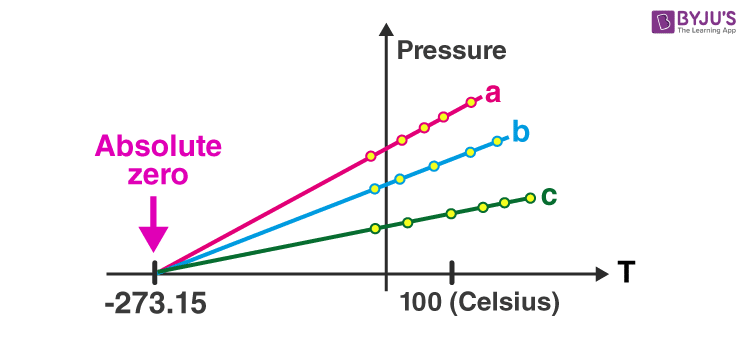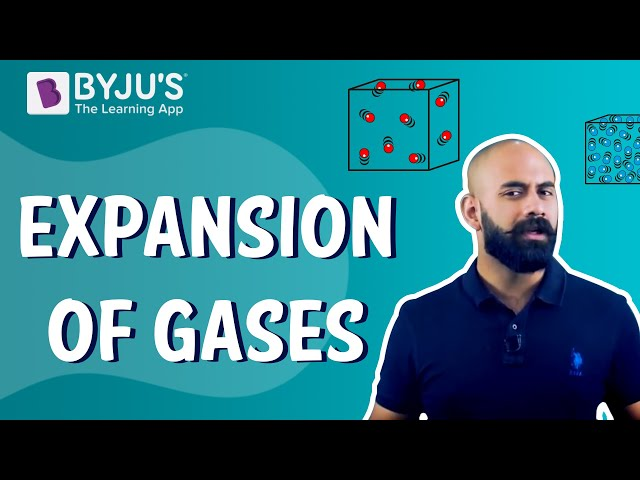# Ideal Gas Law

## What is Ideal Gas Law?

The ideal gas law, also known as the general gas equation, is an equation of the state of a hypothetical ideal gas. Although the ideal gas law has several limitations, it is a good approximation of the behaviour of many gases under many conditions. Benoit Paul Émile Clapeyron stated the ideal gas law in 1834 as a combination of the empirical Charles’s law, Boyle’s Law, Avogadro’s law, and Gay-Lussac’s law.

The ideal gas law states that the product of the pressure and the volume of one gram molecule of an ideal gas is equal to the product of the absolute temperature of the gas and the universal gas constant.

The empirical form of ideal gas law is given by:

 PV=nRT where, P is the pressure V is the volume n is the amount of substance R is the ideal gas constant

### Ideal Gas Law Units

• When we use the gas constant R = 8.31 J/K.mol, then we have to plug in the pressure P in the units of pascals Pa, volume in the units of m3 and the temperature T in the units of kelvin K.
• When we use the gas constant R = 0.082 L.atm/K.mol then pressure should be in the units of atmospheres atm, volume in the units of litres L and the temperature T in the units of kelvin K.

For easy reference, the above information is summarized in the table as follows:

 Ideal Gas equation Units $$\begin{array}{l}R=8.31\frac{J}{K.mol}\end{array}$$ $$\begin{array}{l}R=0.082\frac{L.atm}{K.mol}\end{array}$$ Pressure in pascals Pa Pressure in atmospheres atm Volume in m3 Volume in litres L The temperature in Kelvin K The temperature in Kelvin K

## Derivation of the Ideal Gas Law

The ideal gas law is derived from the observational work of Robert Boyle, Gay-Lussac and Amedeo Avogadro. Combining their observation into a single expression, we arrive at the Ideal gas equation, which describes all the relationships simultaneously.

The three individual expressions are as follows:

Boyle’s Law

$$\begin{array}{l}V\propto \frac{1}{P}\end{array}$$

Charle’s Law

$$\begin{array}{l}V\propto T\end{array}$$

$$\begin{array}{l}V\propto n\end{array}$$

Combining these three expressions, we get

$$\begin{array}{l}V\propto \frac{nT}{P}\end{array}$$

The above equation shows that volume is proportional to the number of moles and the temperature while inversely proportional to the pressure.

This expression can be rewritten as follows:

$$\begin{array}{l}V=\frac{RnT}{P}=\frac{nRT}{P}\end{array}$$

Multiplying both sides of the equation by P to clear off the fraction, we get

$$\begin{array}{l}PV=nRT\end{array}$$

The above equation is known as the ideal gas equation.

### Ideal Gas Solved Problems

1. What is the volume occupied by 2.34 grams of carbon dioxide gas at STP?
Solution:
To determine the volume, rearrange the ideal gas law as follows:

$$\begin{array}{l}V=nRT/P\end{array}$$

Substituting the values as follows, we get

$$\begin{array}{l}V=[(2.34\,g/44\,g.mol^{-1})(0.08206\,{L}\,atm\,mol^{-1}\,K^{-1})(273.0\,K)]/1.00\,atm\end{array}$$

$$\begin{array}{l}V=1.19\,L\end{array}$$

2. Calculate the temperature at which 0.654 moles of neon gas occupies 12.30 litres at 1.95 atmospheres.
Solution:
To determine the temperature, rearrange the ideal gas equation as follows:

$$\begin{array}{l}T=PV/nR\end{array}$$

Substituting the value, we get

$$\begin{array}{l}T=(1.95\,atm))(12.30\,L)/(0.654\,mol)(0.08206\,L\,atm\,mol^{-1}K{-1})\end{array}$$

$$\begin{array}{l}T=447\,K\end{array}$$

## Relationship between Pressure and Temperature

The graph here shows the relationship between temperature and pressure for different gases.On extrapolating this graph, we see that the graphs always intercept the x-axis at a point we now call the absolute zero, irrespective of which gas we are graphing. This point represents the beginning of the Kelvin scale, i.e. Zero K. In the Celsius scale, 0 K is equivalent to -273.5 oC. This is the coldest temperature possible. As a molecule gets colder, its energy and, consequently, its movement and vibrations decrease in amplitude. As we keep cooling it, at a point, the atom will reach a state of minimum internal energy where the atom has lost almost all its energy and is stationary.

### What does the ideal gas state?

The pressure, temperature and volume of a gas are related to each other.

### Who derived the ideal gas?

Benoît Paul Émile Clapeyron derived the ideal gas.

### Why is the ideal gas inaccurate?

The ideal gas only holds true when the conditions at consideration are ideal. Under high pressure and low temperature, the molecular size and the intermolecular forces become important to be considered and are no longer negligible, so essentially the ideal gas law won’t work.

### Does ideal gas law apply to liquids?

No, the ideal gas law doesn’t apply to liquids.

### How is ideal gas law used in everyday life?

Suppose an engineer wants to store 500 g of oxygen in a container kept at a pressure of 1 atm and 125 degrees Fahrenheit. The Ideal Gas Law is used to identify the volume of a container that needs to be built for the same.

## Why does a balloon burst when you bring a candle near it?If you liked the article, download BYJU’S – the learning app to learn more fascinating intricacies of science. Come learn from us and fall in love with learning.

Test your knowledge on Ideal gas law and absolute zero

#### 1 Comment

1. Ranu Beeru Bodekar

It is helpful for my study